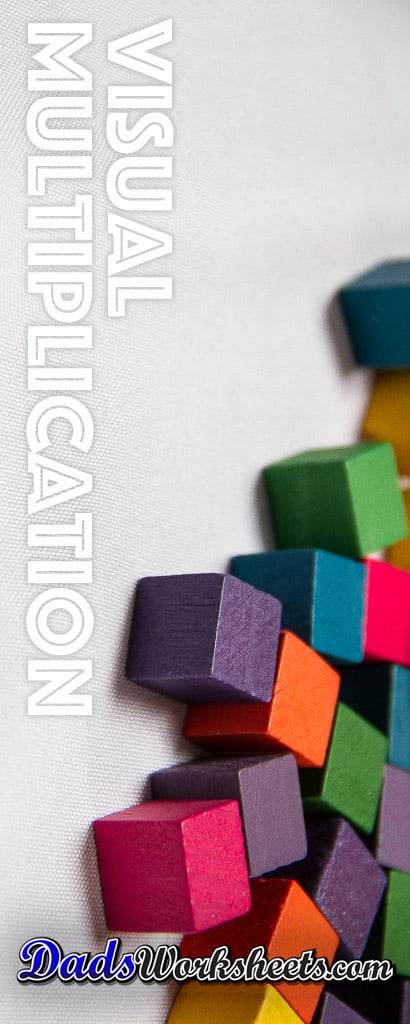# Multiplication Worksheets: Visual Multiplication Worksheets

A good way to start teaching multiplication facts is to provide some sort of visual or manipulative aide to assist with counting strategies for solving basic multiplication facts. These visual multiplication worksheets have arrays of blocks that allow students to count rows, columns and totals to build foundational concepts of how multiplication works.

## Teach Multiplication Concepts Visually!

Multiplication is one of the first math skills where simple counting on ten fingers really doesn't get you very far. For a third grade student to really understand multiplication, they have to develop strategies for dealing with larger numbers, but even before that they have to internalize exactly what multiplication means.

These worksheets are designed to do just that. They are the perfect starting exercise for students who have limited exposure to multiplication and who still rely on visual cues or manipulatives to solve arithmetic problems..

The problems on these worksheets require students to look at the number of rows and columns in an array of manipulatives to determine how many objects are shown in total. By breaking multiplication problems out visually into these two smaller counting exercises, these worksheets can provide a good bridge to skip counting or other multiplication strategies for learning times table facts.

And, I think they're beautiful. All of those images are unique photographs, which gives each multiplication fact a unique look. I hope it makes them feel more tangible for your young mathematicians!